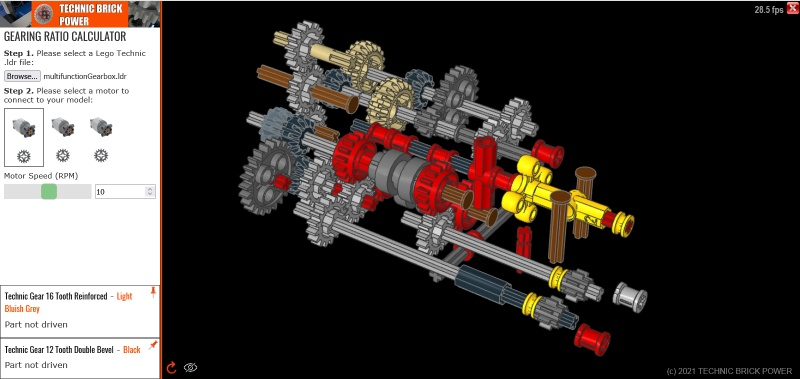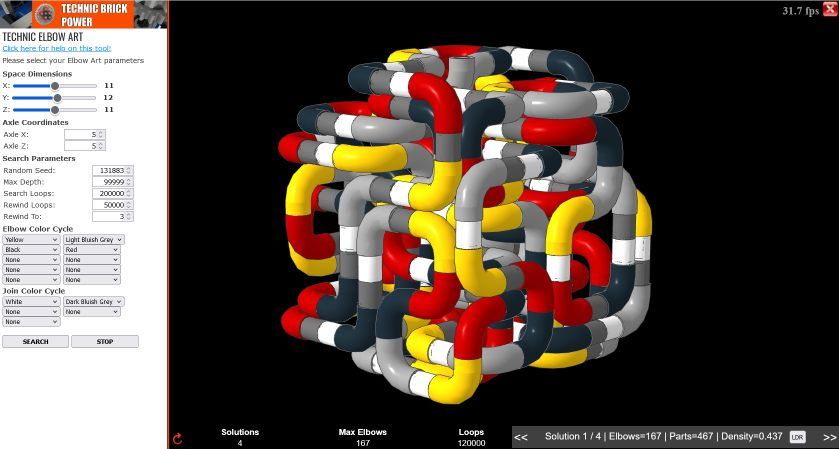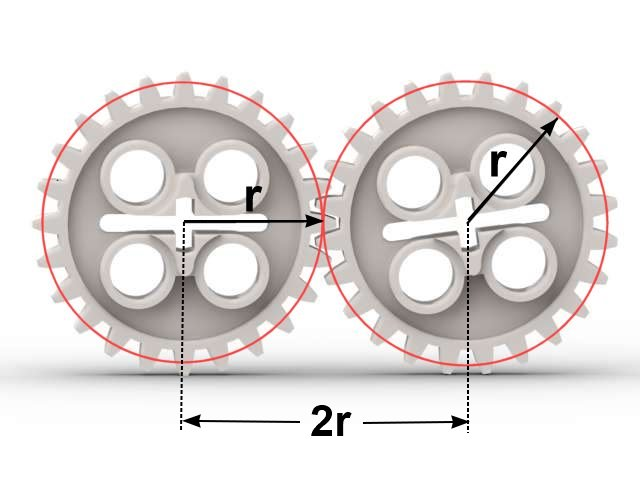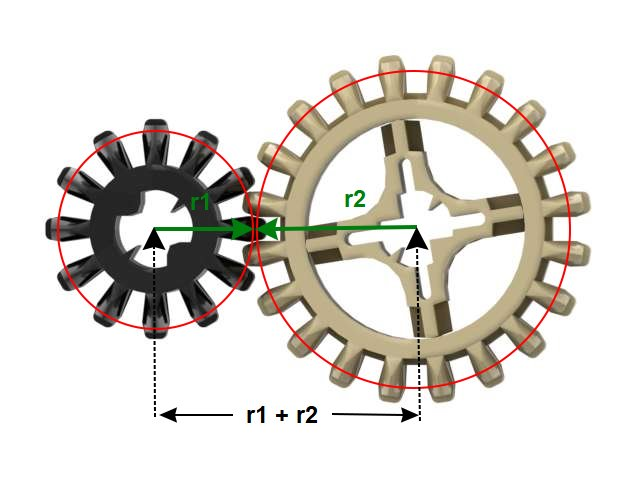# TOOLS

## Gearing Ratio Calculator

Check out the Beta version my Lego Technic Gearing Ratio Calculator Tool:This tool allows you to upload your Lego Technic project .ldr file and analyse the gearing ratios between any two connected gears or axles. Currently supports most gears, axles, worm gears, some differentials, cv joints and universal joints. See the video tutorial on how to use it. It is a work in progress and I would love to hear your feedback on it. Click here to try it out.

Note: As of 25 Septemeber 2021 the tool supports CV joints, linear speed via the radius of the component and improves on the frame rate for higher numbers of parts in a model).

## Technic Elbow Art

This is a tool to create "Lego Technic Elbow Art" - a series of intertwined elbow pieces as a single sequence. Choose your dimensions and search parameters and search for new dense solutions and set a record!# What is the relationship between the number of teeth on a gear and it's radius?

19 Sep 2021

Great question! In order for two Lego Technic gears to mesh their teeth spacing must be the same - therefore all Lego gears (that mesh) have the same teeth spacing. Now you can think of a gear as a circle with a number of teeth fitted around the outside (imagine a string of teeth wrapped around a circle) and therefore the number of teeth that fit around the circle is just the circumference of the circle divided by the width of the teeth. Of course in order for two gears to mesh and drive each other the teeth have to overlap between the gears as shown in the diagram below. The red circles are known as the pitch circles representing the distance from the center of each gear such that the pitch circles of meshing gears touch. The pitch circles have a pitch radius of r which is also sometimes described by a pitch diameter. In the case of same sized gears that means the pitch circles are the same size and the distance between the centers of the gears is simply 2r (two times the radius of the pitch circle) or simply the pitch diameter.Now the circumference of a circle is related to the radius of the circle by the well known formula 2 x pi x r where r is the radius of the circle. Since this is a linear relationship it means that if for example you double the radius of a gear then the number of teeth around it will double as well.  So a 40 tooth gear will have two times the radius of a 20 tooth gear.

When the Lego company first designed the Lego gears they had to choose a tooth sizing that would work well and they decided to choose a sizing such that:

Pitch Radius = Gear Teeth / 16 (in Lego units)

So to work out the pitch radius of a gear you just divide the number of teeth on the gear by 16. So for example a 24 tooth gear has a pitch radius of 24 / 16 = 1.5.

Since almost all Lego Gears have teeth with multiples of 4 it means that the pitch radius of most gears are multiples of 0.25 (1/4), e.g. 1.25, 1.5, 1.75, 2.0, 2.25 etc.

What makes the pitch radius important is that the distance between the centers (or axles) of two meshing gears is simply the sum of the pitch radii of the two gears and it is this fact allows you to work out the required distance between any two gears in order to mesh them correctly. The equation is:

axle spacing = r1 + r2

where r1 and r2 are the pitch radii of the gear 1 and gear 2 respectively. Combining this equation with the one above gives:

axle spacing = (t1 + t2) / 16

where t1 and t2 are the number of teeth on gear 1 and gear 2.

For example consider the following common Lego Technic meshing of a 12 tooth gear driving a 20 tooth gear:We have t1 = 12 and t2 = 20, therefore the axle spacing is (12 + 20) / 16 = 2 which is simply a spacing of two units on a liftarm. Now generally it is not easy or convenient to create fractional spacing, e.g. 1.5 or or 1.75 so to easily mesh two gears on a regular spacing the sum of their teeth must be a multiple of 16. Some the well known combinations are 8 + 8,  8 + 24, 16 + 16, 12 + 20,  24 + 24, 20 + 28, 12 + 36, 8 + 40, 28 + 36, 24 + 40, 8 + 56. To mesh other combinations of gears more advanced techniques are required.

# More to explore

## Lego Technic Gearing Ratio Calculator Tool

16 Jul 2021

Here I present my new Lego Technic Gearing Ratio Calculator tool. This tool allows you to upload your Lego Technic .ldr (Lego Draw) file and it will simulate the gearing system and visualize it as well as showing the gearing ratios relative to the input component.

The tool can be accessed...read more

## How to mesh any two Lego gears on a regular grid!

22 Aug 2020

In this video I analyze all of the eight regular lego technic gears and demonstrate how any two of them can be meshed on a lego grid.

A sum of radii chart is used to determine the possible diagonal meshing combinations. These are then combined with "intermediate" or "idler&...read more

## Lego Technic PU 2 Speed Automatic Gearbox

8 Jul 2022

This video presents a lego technic two speed automatic gearbox using two motors and the Powered Up (PU) lego technic control system. Each motor uses a pivoting gear to either connect to it's gear to the left when rotating in the forward direction or to the gear to the right when reversing. Th...read more

## New Flat 12/20 Gear Friction

23 Apr 2022

The Lego Technic set 42140 App-Controlled Transformation Vehicle introduces two new gears - a flat version of the 12 tooth bevel gear and a flat version of the 20 tooth bevel gear. These gears are designed to have less friction.

In this video I measure the difference in gearing efficiency...read more

## Measuring Gearbox Efficiency

6 Nov 2021

This video describes a method for measuring the efficiency of a gearbox using the Lego Technic Powered Up hub. Gearbox efficiency is defined as the ratio of the output power to the input power and here I show how to measure each of these values to allow you to calculate the overall efficiency.read more

## Lego Technic Gearing Ratio Tool Update

4 Sep 2021

This video presents updates to the Technic Brick Power Lego Technic Gearing Ratio Calculator tool specifically on underconstrained differential systems. These are systems in which the differentials are not fully constrained and therefore have 1 or more degrees of freedom within them.